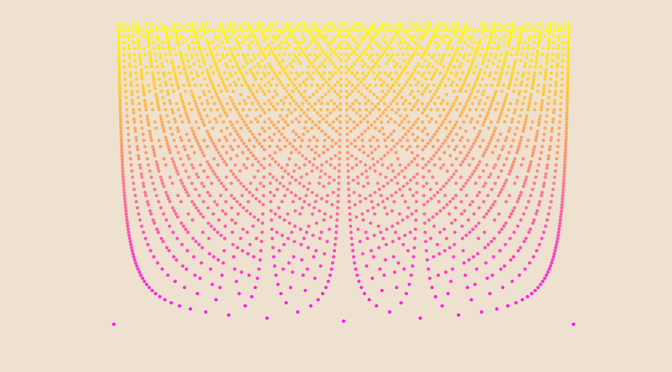A nowhere locally bounded function

In that article, I described some properties of Thomae’s function$$f$$. Namely:

• The function is discontinuous on $$\mathbb{Q}$$.
• Continuous on $$\mathbb{R} \setminus \mathbb{Q}$$.
• Its right-sided and left-sided limits vanish at all points.

Let’s modify $$f$$ to get function $$g$$ defined as follow:
$g: \left|\begin{array}{lrl} \mathbb{R} & \longrightarrow & \mathbb{R} \\ x & \longmapsto & 0 \text{ if } x \in \mathbb{R} \setminus \mathbb{Q} \\ \frac{p}{q} & \longmapsto & q \text{ if } \frac{p}{q} \text{ in lowest terms and } q > 0 \end{array}\right.$ $$f$$ and $$g$$ both vanish on the set of irrational numbers, while on the set of rational numbers, $$g$$ is equal to the reciprocal of $$f$$. We now consider an open subset $$O \subset \mathbb{R}$$ and $$x \in O$$. As $$f$$ right-sided and left-sided limits vanish at all points, we have $$\lim\limits_{n \to +\infty} f(x_n) = 0$$ for all sequence $$(x_n)$$ of rational numbers converging to $$x$$ (and such a sequence exists as the rational numbers are everywhere dense in the reals). Hence $$\lim\limits_{n \to +\infty} g(x_n) = + \infty$$ as $$f$$ is positive.

We can conclude that $$g$$ is nowhere locally bounded. The picture of the article is a plot of function $$g$$ on the rational numbers $$r = \frac{p}{q}$$ in lowest terms for $$0 < r < 1$$ and $$q \le 50$$.